# Plot GPR data

Note:

• This R-package is still in development, and therefore some of the functions may change in a near future.
• If you have any questions, comments or suggestions, feel free to contact me (in english, french or german): .

# Objectives of this tutorial

• Learn how to plot GPR data.

## Install/load RGPR

# install "devtools" if not already done
if(!require("devtools")) install.packages("devtools")
devtools::install_github("emanuelhuber/RGPR")
library(RGPR)       # load RGPR in the current R session


## The GPR data

RPGR comes along with a GPR data called frenkeLine00. Because this name is long, we set A equal to frenkeLine00:

x <- frenkeLine00
x

## *** Class GPR ***
##  name        = LINE00
##  filepath    = data-raw/LINE00.DT1
##  1 fiducial(s)
##  description =
##  survey date = 2014-04-25
##  Reflection, 100 MHz, Window length = 399.6 ns, dz = 0.4 ns
##  223 traces, 55.5 m
##  ****************


# Plot the GPR data

### Raster plot

To plot the GPR record as a raster image (default mode), enter

plot(x)The green line indicates the position of time-zero. The yellow triangle indicates the position of a fiducial marker that was set during the survey to mark something (such as a specific object close to the GPR line, a change in morphology/topography/sedimentology or an intersection with another GPR line). These markers are very useful to add topographic data to the GPR profile, particularly when the fiducial markers correspond to the locations where the (x,y,z) coordinates were measured.

Do you want another color palette? RGPR comes with predefined color palettes. Check them with:

displayPalGPR()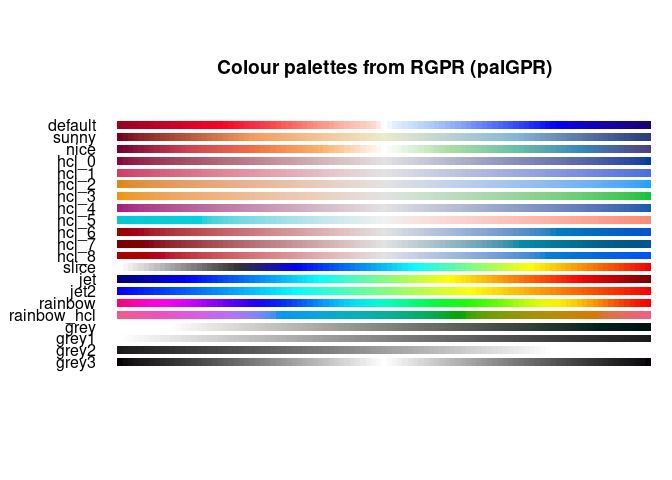Plot a single palette:

plotPal(palGPR("nice"))Choose the color palette you want and plot the GPR data with it:

plot(x, col = palGPR("nice"))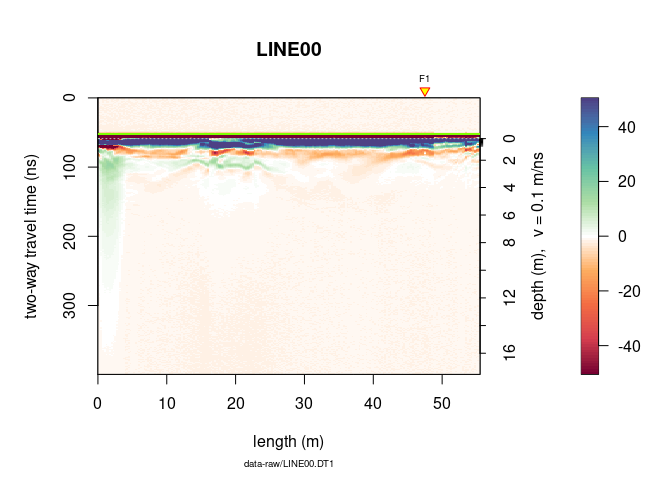You can reduce the number of colors with:

plot(x, col = palGPR("nice", n = 5))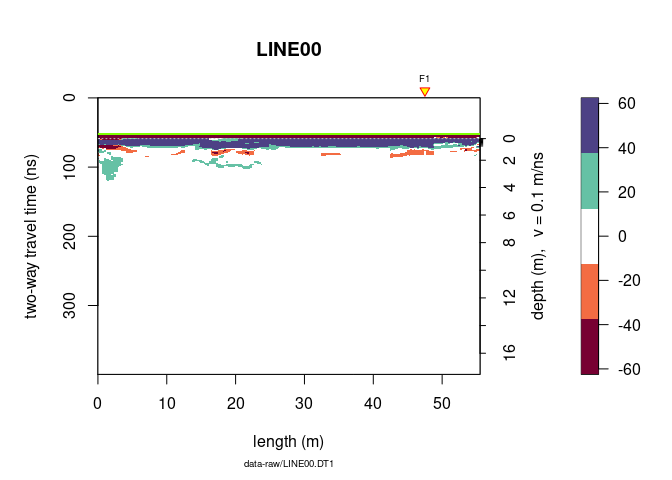### Wiggle plot

Plot wiggles

plot(x, type = "wiggles")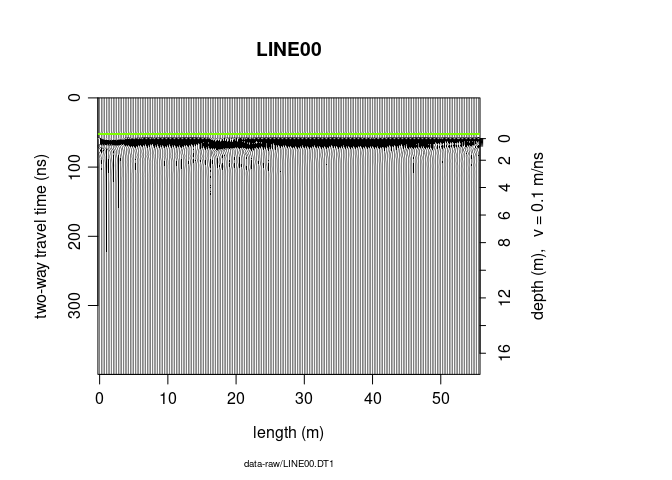### Plot settings

#### Set x and y limits

To plot only a part of the GPR data, use xlim and ylim.

plot(x, ylim = c(50, 100), xlim = c(30, 40))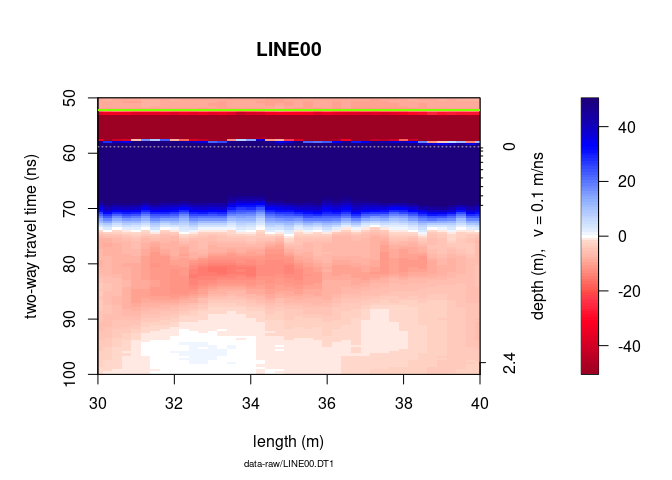Another way to plot only a part of the GPR data is to extract a part of the GPR data. The object A can be manipulated in the same way as a matrix without losing the meta-data (e.g., trace coordinates, antenna separation).

To plot only the samples 100 to 300 of the $15^{th}$ to $150^{th}$ :

# plot the 100 to 300 samples of the traces 15 to 150
plot(x[100:300, 15:150])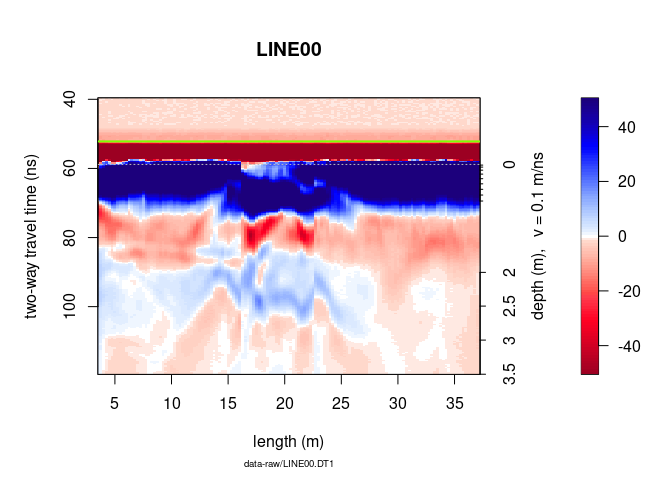#### Change the scale of the colorbar

To change the span of the colorbar from -10 to 25 do

plot(x, zlim = c(-10, 25))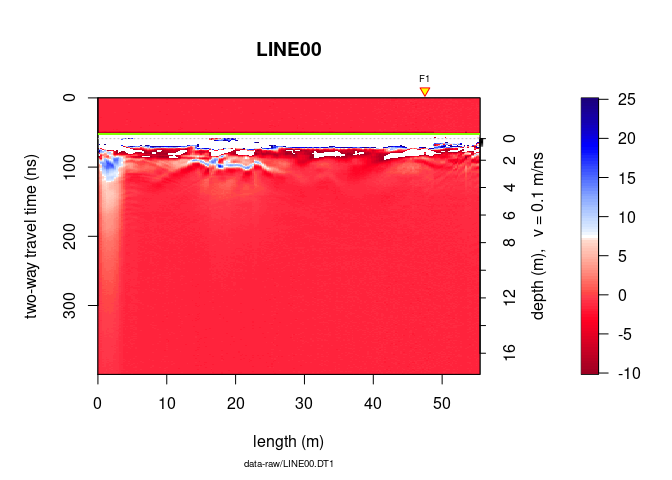#### Display GPR data relative to time-zero

To set the origin of the vertical axis at time-zero, set the argument relTime0 equal to TRUE.

plot(x, relTime0 = TRUE, ylim = c(0, 200), xlim = c(30, 50))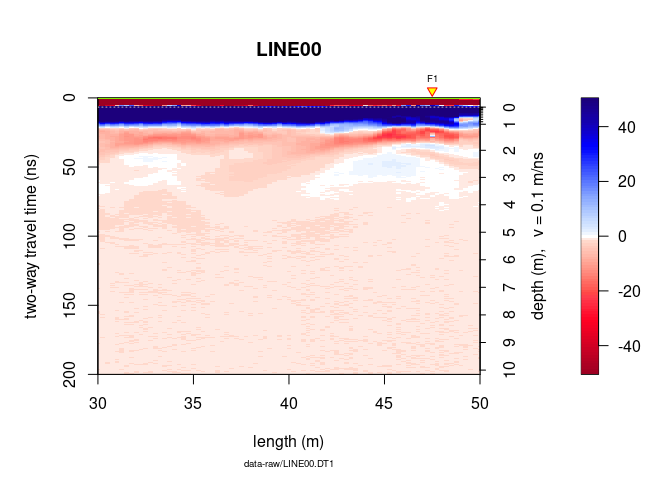#### Markers, annotations, note…

To not display the markers, the annotation (e.g., markers for the intersection of the GPR line with other GPR lines), the time-zero line, the colorbar (barscale), set addFid, addAnn, addTime0 and barscale equal to FALSE.

plot(x, addFid = FALSE, addAnn = FALSE, addTime0 = FALSE, barscale = FALSE)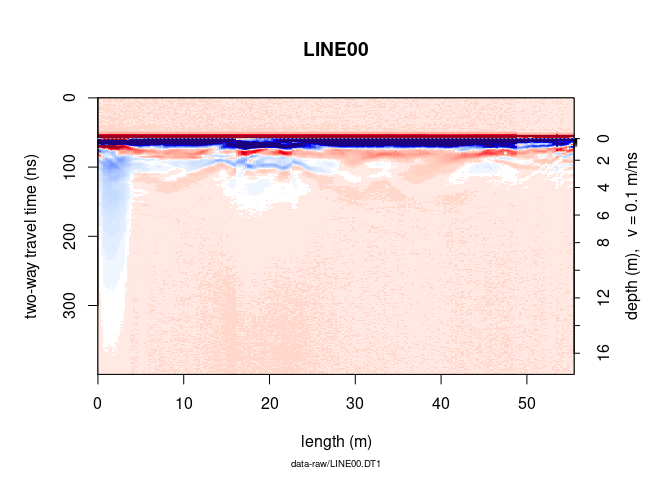To not display the note below the x-label, the plot title (file name), the x and y lables, the colorbar label, set note, main, ylab, xlab and clab equal to "".

plot(x, note = "", main = "", ylab = "", xlab = "", clab = "")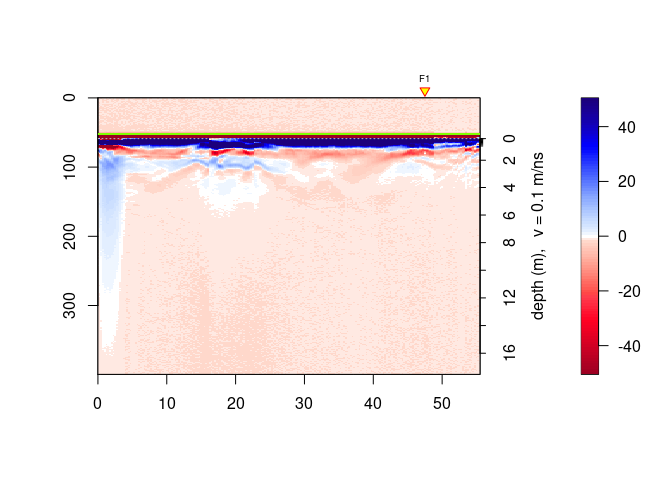### ggplot2 plots

Plots with ggplot2, a R-package based on the Grammar of Graphics.

x <-frenkeLine00

ggplotGPR(x)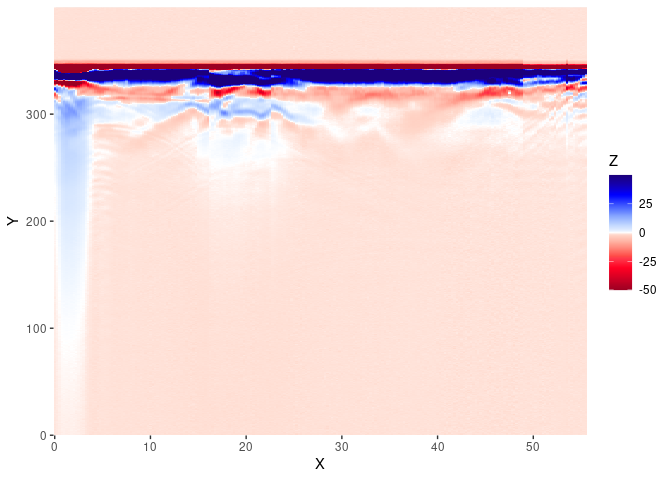The ggplotGPR() function is a wrapper for:

col <- palGPR()
x <- frenkeLine00

Xm <- data.frame(x = rep(pos(x), nrow(x)),
y = rep(depth(x), each = ncol(x)),
value =  as.vector(t(as.matrix(x)[nrow(x):1, ])))
ggplot2::ggplot(Xm) +
ggplot2::geom_tile(ggplot2::aes(x = x, y = y, fill = value)) +
ggplot2::scale_x_continuous("X", expand = c(0, 0)) +
ggplot2::scale_y_continuous("Y", expand = c(0, 0)) +


### Interactive plots

Use the function plotly() to produce interactive plots powered powered by the JavaScript library plotly.js. The function plotly() works only with 2D data.

x <- frenkeLine00

plotly(x)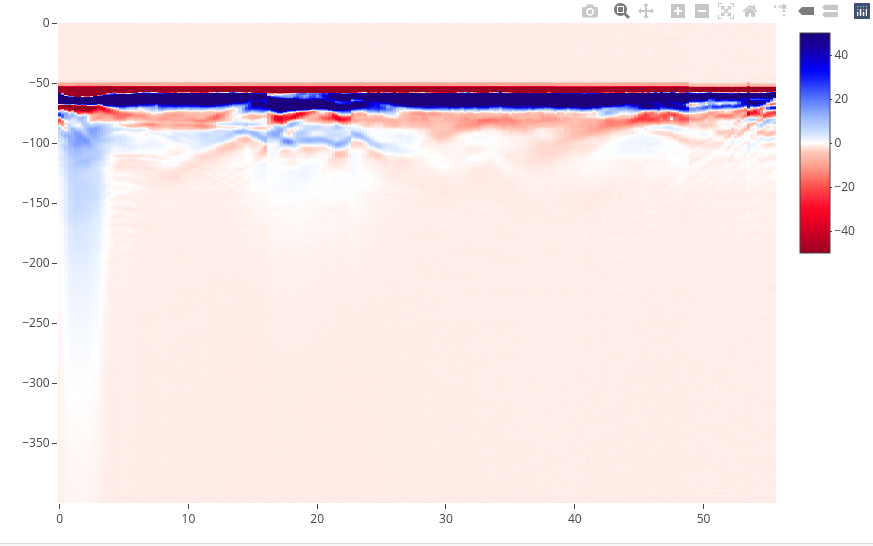### Multi-plots

Use par(mfrow = c(nr, nc)), where ncr is the number of rows and nc is the number of column. Example:

x <- frenkeLine00

par(mfrow = c(2,2), oma = c(0, 0, 0, 0))
plot(x)
plot(x, col = palGPR("nice"))
plot(x, col = palGPR("sunny"))
plot(x, col = palGPR("hcl_0"))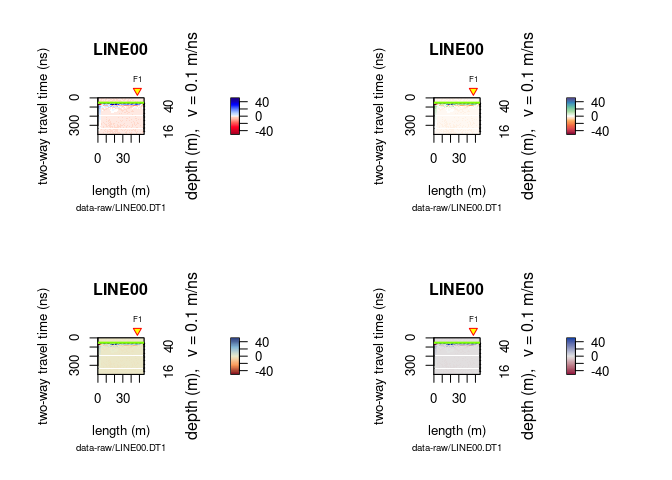### Annotate plots

Here an example on how to annotate plots:

x <- frenkeLine00

plot(x)
rect(xleft = 35,
ybottom = 120,
xright = 45,
ytop = 50,
border = "firebrick",
lty = 3,   # line style: 1 = continuous line
lwd = 2)   # width
# text annotation
text(45, 50, "A20-21Z", adj = c(0, 0))
#grid
grid(col = "red")
# point
points(50, 300, pch = 21, col = "red", lwd = 2)
# horizontal and vertical linges
abline(h = 300, v = 30, col = "black", lty = 2, lwd = 3)

# For circles, squares and stars the units of the x axis are used
# circles
symbols(30, 300, circles = 10,
add = TRUE, lwd = 2, fg = "dodgerblue", inches = FALSE, lty = 1)
# rectangles
symbols(5, 200, rectangles = matrix(c(5, 200), nrow = 1, ncol = 2),
add = TRUE, lwd = 3, fg = "dodgerblue", inches = FALSE, lty = 3)
# stars
symbols(20, 150, stars = matrix(c(0.35, 1, 0.35, 1,  0.35, 1,  0.35, 1, 0.35, 1), nrow = 1),
add = TRUE, lwd = 1, bg = "goldenrod1", fg = "black", inches = FALSE, lty = 1)## One-dimensional plot

### Single trace plot

Plot a signal trace, notice that the signal is clipped to $\pm50\,mV$ (between $0$ and $20\,ns$ ):

plot(x[, 15])      # plot the 15th trace of the GPR-line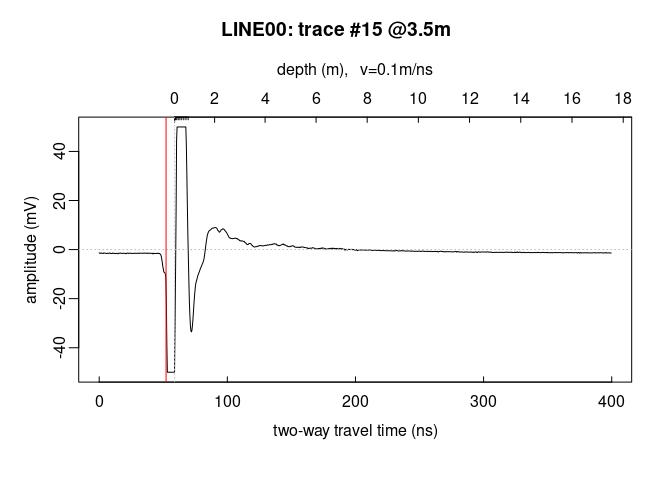Note: the @3.5m in the plot title indicate the relative position of the trace on the GPR profile.

To add another trace, use the function lines()

plot(x[, 15])      # plot the 15th trace of the GPR-line
lines(x[, 16], col = "red", lwd = 2)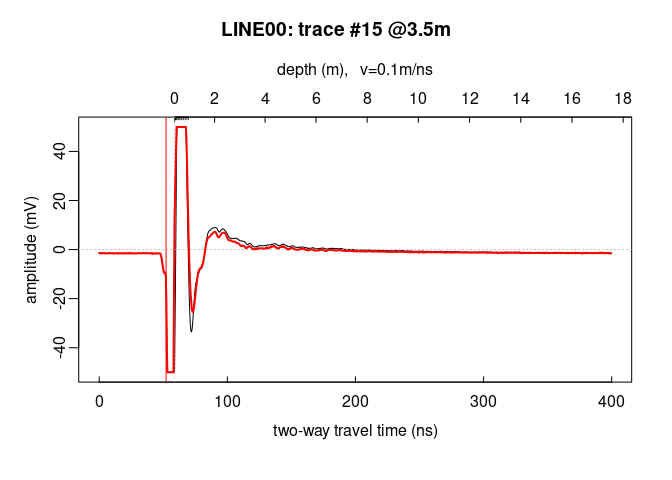Plot the first 40 trace samples:

# plot the first 40 samples of the 15th trace of the GPR profile
plot(x[1:40, 15])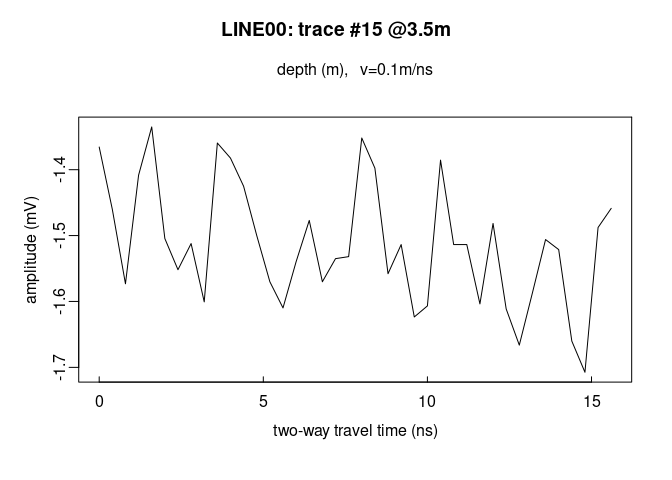### Multiple trace plot

Plot all the traces within a single plot:

trPlot(x, col = rgb(0.2, 0.2, 0.2, 7/100))     # plot all the traces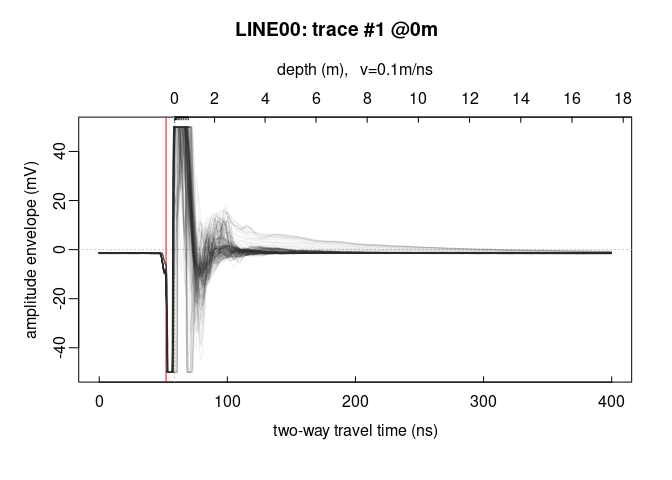trPlot(x, col = rgb(0.2, 0.2, 0.2, 7/100))     # plot all the traces
lines(traceStat(x), lwd = "2", col = "red")## Frequency plots

### Frequency/Phase-Amplitude (1D)

Let’s have a look at the amplitude-frequency and phase-frequency plot (the spectrum given by the Fourier decomposition):

spec(x)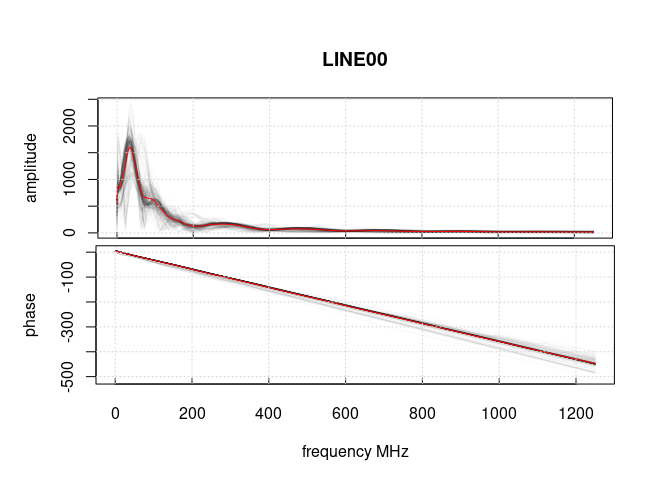## Frequency-wavenumber filter (f-k-filter)

The function spec() with the argument type = "f-k returns a list containing the frequencies (f), the wavenumbers (k), the amplitude of the GPR data.

spec(x, type = "f-k")Check the help for more details on the plot() function:
?plot.GPR